# Gentzen formal system

(diff) ← Older revision | Latest revision (diff) | Newer revision → (diff)

A logical calculus which serves for the formalization and study of meaningful proofs that operate by introduction and discharge of hypotheses. Introduced by G. Gentzen . Gentzen formal systems are subdivided into systems of natural derivations (or natural deduction systems, which imitate the form of ordinary mathematical derivations, and are especially well suited to putting the latter in formal notation) and sequent systems (or logistic systems, which allow the survey of possible proofs of a given formula, obtain results on the normal form of a proof, and their application to proof theory, and to the theory of automated theorem proving). Gentzen formal systems are sometimes identified with systems of sequent type; nevertheless, natural deduction systems may employ sequents (cf. Sequent (in logic)), while sequent Gentzen formal systems are sometimes formulated as calculi of formulas rather than of sequents; all Gentzen formal systems are sometimes treated as natural deduction systems, since they reflect to some extent the usual methods of handling logical connectives and assumptions.

Natural deduction systems comprise rules for the introduction of logical symbols and their discharge. There are few logical axioms (usually one or two). For instance, the natural variant of classical propositional calculus for the languageis defined by the axiom; by the introduction rules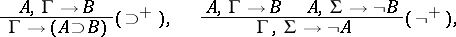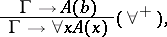wheredoes not occur inor; by the elimination rules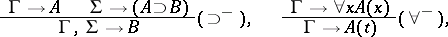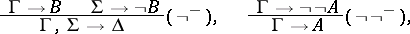whereis an arbitrary term; and by structural rules: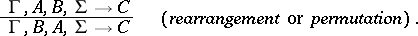The sequent written below the line is said to be the conclusion of the rule, while the sequents above the line are called premises. The axiom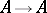reflects the introduction of the assumption; the ruleillustrates the discharge of an assumption: the formulaof the upper sequent depends on the assumption, but the formula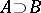of the lower sequent no longer depends on. A natural deduction system is occasionally defined as a calculus of formulas (as distinct from calculi of sequents) with an implicit notation of dependence on the assumptions: a derivation in such a calculus is a tree-like graph, at the vertices of which arbitrary formulas (not necessarily axioms) can be situated, and all the transitions proceed according to derivation rules. These rules are obtained by deleting the antecedents from the respective rules of the natural system described by sequents; discharge of an assumption involves the addition of appropriate conditions; for example,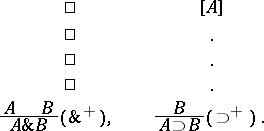It is considered that an occurrenceof a formula in such a derivation depends on an assumption that, unlessis an axiom, is found at a vertex of the derivation aboveand the assumptionis not discharged in the branch leading from the occurrence ofunder consideration towards. In interpreting a derivation of this kind each occurrence of a formulais transformed to a sequent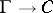, whereis a complete list of assumptions on which the relevant occurrence of the formuladepends. The connection between natural deduction systems and ordinary (Hilbertian) variants of the corresponding systems is established using the following statement:is deducible in the natural deduction system if and only ifis deducible fromwith fixed variables in an ordinary system.

Natural deduction systems in their original form are not very suitable for the analytical search for a derivation: attempts to determine the premises and the rules from which a given formula (sequent) may be deduced lead to ambiguities — in principle, this may be the rule for the introduction of the appropriate logical connective or any elimination rule. The set of possible premises in the elimination rules is potentially unlimited (by varying the formulain the rule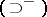). It is useful, for this reason, to have rules with the subformula property: The premises contain only the subformulas of the conclusion, while the infinite number of possibilities only occurs in the choice of terms in the rules of the type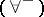. In sequent Gentzen formal systems all formulas have the subformula property or else this property is violated for one rule only — the cut rule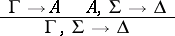or another similar rule, e.g. for the rule. Gentzen formal systems with the subformula property are also called cut-free Gentzen formal systems for this reason.

### Example.

A cut-free variant of the classical predicate calculus LK. The deducible objects are arbitrary sequents, consisting of formulas in. The axiom is.

Succedent rules: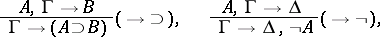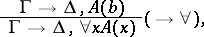wheredoes not occur inand.

Antecedent rules: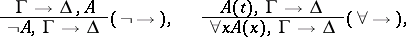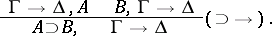Structural rules: cut, rearrangement, refinement and contraction in the antecedent and in the succedent (cf. Sequent (in logic)).

In comparing sequent and natural deduction systems, succedent rules correspond to introduction rules, while antecedent rules correspond to elimination rules. The cut is employed in modelling the elimination rules in sequent Gentzen formal systems. The subformula property for LK is ensured by Gentzen's theorem (the cut-elimination theorem): For each derivation in LK it is possible to construct a cut-free derivation (of the same sequent). This theorem makes it possible to establish the solvability of quantifier-free systems: using subformulas of a given quantifier-free formula it is possible to construct only a finite number of matching sequents (sequents are matching if they differ only in the order and in the repetitions of terms in the antecedent and in the succedent), from which it is possible in turn to construct only a finite number of "candidates" to yield derivations; the given formula is provable if such candidates include a derivation.

Gentzen formal systems make it possible to express meaningful features of theories with the aid of purely-constructive concepts; thus, a Gentzen formal system of intuitionistic mathematics often differs from the corresponding classical system only in that mono-succedent rather than arbitrary sequents are employed. In cut-free systems consistency (i.e. non-deducibility of the empty sequent) and the deducibility of one of the disjunctive terms of a disjunction (in the intuitionistic case) being deduced are readily shown.

An important generalization of Gentzen formal systems are semi-formal systems containing rules with an infinite set of premises, such as the rule of infinite induction (-rule):How to Cite This Entry:
Gentzen formal system. Encyclopedia of Mathematics. URL: http://encyclopediaofmath.org/index.php?title=Gentzen_formal_system&oldid=13610
This article was adapted from an original article by G.E. Mints (originator), which appeared in Encyclopedia of Mathematics - ISBN 1402006098. See original article